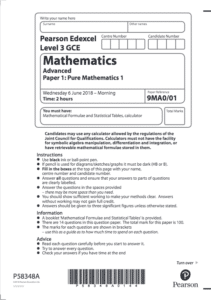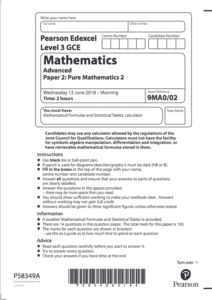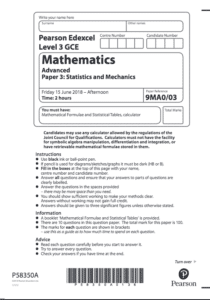# A2 Maths (second year of A-Level Maths)

A2 Maths is the name we give to the second year of A-Level Mathematics. For the full A-Level in Maths, students must study for two full years and take exams in the summer at the end of Year 2. Students also have the option to get a qualification in AS Maths for which they will only study for one year (see more on AS Maths). However, there are more exams and they are more advanced in content and difficulty when studying both the AS and A2 Maths years. See below for more exam information or click here to see the Edexcel website information for A-Level Mathematics.

## Topics covered in A2 Maths

Pure Maths:

Statistics:

1. Statistical Sampling: (not covered in A2 Maths)
2. Data Presentation & Interpretation: (not covered in A2 Maths)
3. Probability: conditional probability
4. Statistical Distributions: the normal distribution
5. Statistical Hypothesis Testing: more hypothesis testing

Mechanics:

1. Quantities & Units in Mechanics: (not covered in A2 Maths)
2. Kinematics: 2D kinematics
3. Forces & Newton’s Laws: 2D forces
4. Moments: moments

## A-Level Maths Exam Information

From September 2017, the Edexcel Maths A-Level will be taught from a new linear syllabus. Students will start learning the material in September and will take two exams in May/June two years later. The examinations are made up of 3 papers: 2 papers on Pure Mathematics and 1 paper on Statistics and Mechanics. Students are expected to be familiar with the Large Dataset.

Paper 1:Paper 1 is the first paper that covers the pure (or core) aspects of the course. The paper is out of 100 marks and is worth 33.33% of the qualification. The duration is 2 hours and the exam paper code is 9MA0/01.

• Paper examines Pure Maths components
• Out of a possible 100 marks
• Worth 33.33% of the A-Level Maths qualification
• Duration – 2 hours
• Paper code – 9MA0/01

Paper 2:Paper 2 is the second paper that covers the pure (or core) aspects of the course. The paper is out of 100 marks and is worth 33.33% of the qualification. The duration is 2 hours and the exam paper code is 9MA0/02.

• Paper examines Pure Maths components
• Out of a possible 100 marks
• Worth 33.33% of the A-Level Maths qualification
• Duration – 2 hours
• Paper code – 9MA0/02

Applied Maths: Papers 3+4:Papers 3 and 4 cover the applied (statistics and mechanics) aspects of the course. Each paper is worth 50 marks in total and combined are worth 33.33% of the qualification. The duration is 2 hours and the exam paper codes are  9MA0/31 and 9MA0/32.

• Papers 3 and 4 examine Statistics and Mechanics components
• You are given both papers and given 2 hours to complete them both. They are out of a possible 100 marks.
• Worth 33.33% of the A-Level Maths qualification
• Duration – 2 hours
• Paper codes – 9MA0/31 (Stats) and 9MA0/32 (Mech.)

(Updated July 2022)